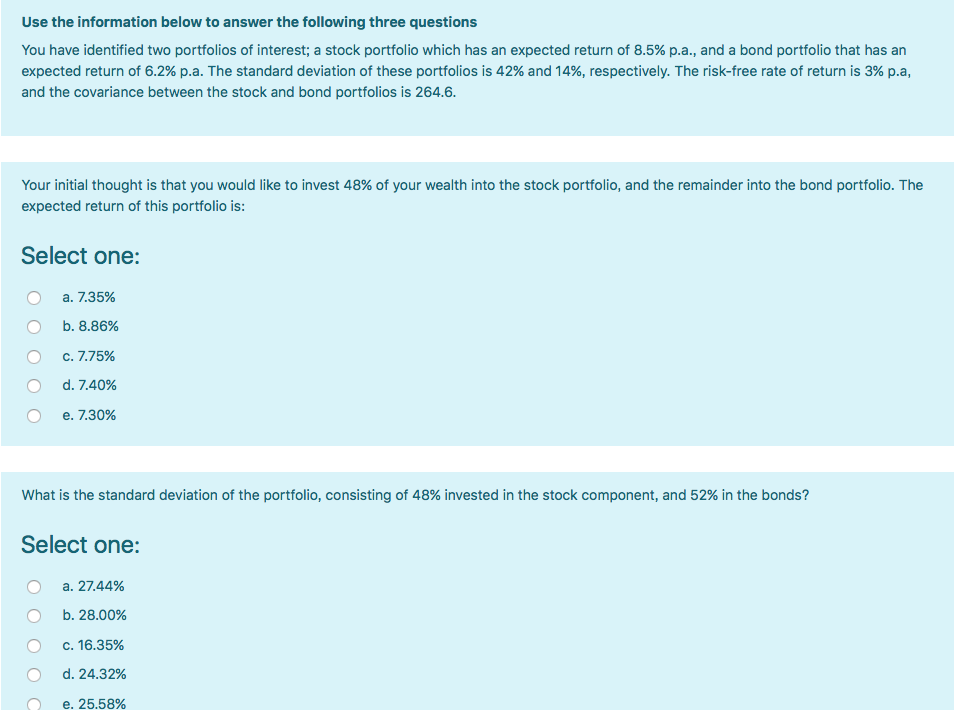### Create an Account

Home / Questions / Use the information below to answer the following three questions

# Use the information below to answer the following three questionsUse the information below to answer the following three questions You have identified two portfolios of interest; a stock portfolio which has an expected return of 8.5% p.a., and a bond portfolio that has an expected return of 6.2% p.a. The standard deviation of these portfolios is 42% and 14%, respectively. The risk-free rate of return is 3% p.a, and the covariance between the stock and bond portfolios is 264.6. Your initial thought is that you would like to invest 48% of your wealth into the stock portfolio, and the remainder into the bond portfolio. The expected return of this portfolio

Jan 31 2020 View more View LessGet Solution